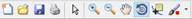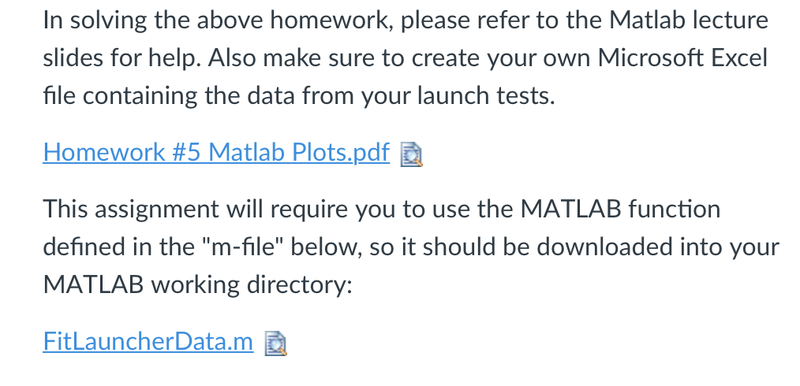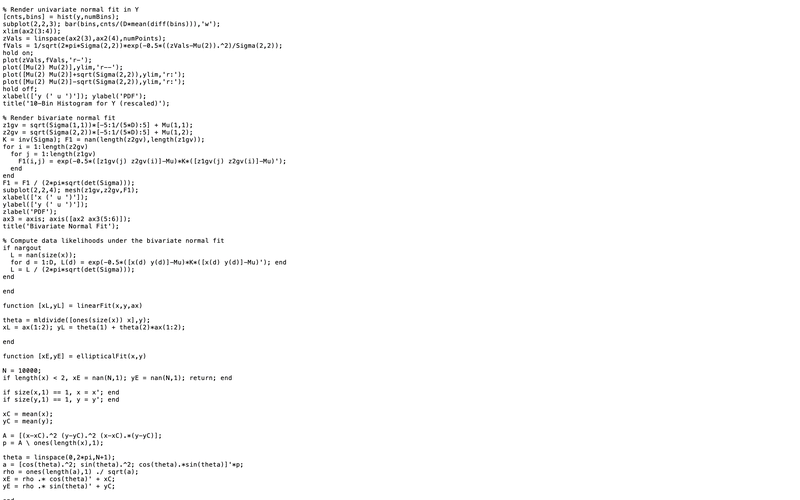# We Helped With This Electrical Engineering Assignment: Have A Similar One?

SOLVEDCategory Engineering Electrical Engineering Undergraduate Solved Electrical Engineering Assignment Help

## Assignment Description

EGN 1001 – Introduction to Engineering

Homework #5 – Matlab Plotting & Accuracy/Precision

Assigned:  February 11, 2019             Due:  February 18, 2019

1) Matlab Assignment:

a.      Using the (X,Y) data collected from your Launcher, generate the following plots using Matlab:

i.        2D Line plots (you may find the command plot useful)

1.      X vs. Launch #

2.      Y vs. Launch # ii. 2D Bar plots (you may find the command bar useful)

1.     X vs. Launch #

2.     Y vs. Launch # iii. 2D Scatter plot, or plotting Y vs. X without connecting the points (see the linestyle options of the plot command)

b.      Using the (X,Y) data collected from your Launcher, calculate the following in Matlab (you may find the commands mean and std useful):

i.        The mean (average) and standard deviation (spread) of the X measurements alone

ii.      The mean (average) and standard deviation (spread) of the Y measurements alone

c.       A file called FitLauncherData.m is provided with this assignment. Use MATLAB’s help command to determine appropriate use of the function for your specific data.

i.        Successful use of the function will render four plots into a single figure window, one of them being a 3D “bell curve” approximation of your 2D data. Select the “Rotate 3D” tool in the

figure windowand then drag the mouse over the bell curve to

view it from the top, aligning its x and y axes perfectly with the x and y axes of the original scatter plot. Explain the elliptical fits of your data implied by the 3D bell curve in terms of the means and standard deviations calculated in part (b). What additional information is provided by the 3D bell curve that is not apparent from the curves that approximate the x data alone and the y data alone?

ii.      Use the function to also compute the so-called “likelihoods” of your data. Then, augment the rendered 3D bell curve to show your (X,Y) data plotted along the bell’s surface. Hint: Employ MATLAB’s plot3 function (without connecting the points), but within hold commands so that the bell curve is not overwritten e.g., at the MATLAB prompt, type

hold on; plot3(<insert appropriate arguments>); hold off; d. Deliverable:

i.          A document with a paragraph commenting on the accuracy and the precision of your launcher (use parts (a), (b) and (c) from above to justify your conclusions)

ii.        Include all plots generated above, each with axes labeled and an appropriate title (e.g., employ the xlabel, ylabel and title commands), in your document.

iii.      Copy and paste your command window session from Matlab and include it with the report

(please format this text for easier reading, also removing commands that generated errors).

e. Select one group member’s graphs and comments to be printed and added to your lab report.

 You are welcome to inspect the code inside this function but appreciate that it is beyond our course’s scope both mathematically and MATLAB-wise. Its purpose here is only to stimulate thought on the extent to which your launcher data is appropriate for ``bell curve” assumptions (called the normal, or Gaussian, distribution in mathematics, in which ellipsoids plays an important role).

## Assignment ImageIn solving the above homework, please refer to the Matlab lecture slides for help. Also make sure to create your own Microsoft Excel file containing the data from your launch tests. Homework #5 Matlab Plots.pdf This assignment will require you to use the MATLAB function defined in the "m-file" below, so it should be downloaded into your MATLAB working directory: FitLauncherData.m

## Assignment Image% Render univariate normal fit in Y [cnts, bins] = hist (y, numBins); subplot (2,2,3); bar (bins, cnts/(D*mean (diff(bins))), 'w'); xlim (ax2 (3:4)); zVals = linspace(ax2 (3), ax2 (4), numPoints); fVals = 1/sqrt(2*pi*Sigma (2, 2)) *exp(-0.5*((zVals-Mu (2)).^2)/Sigma (2, 2)); hold on; plot (zVals, fVals, 'r-'); plot([Mu (2) Mu(2)],ylim, 'r--'); plot([Mu (2) Mu (2)]+sqrt(Sigma (2, 2)), ylim, 'r: '); plot([Mu (2) Mu (2)]-sqrt(Sigma (2, 2)), ylim, 'r: '); hold off; xlabel(['y (' u ')']); ylabel('PDF'); title('10-Bin Histogram for Y (rescaled)'); % Render bivariate normal fit z1gv = sqrt(Sigma (1,1))* [-5:1/(5*D): 5] + Mu(1,1); z2gv = sqrt(Sigma (2, 2))* [-5:1/(5*D):5] + Mu (1, 2); K = inv (Sigma); F1 = nan (length(z2gv), length (z1gv)); for i=1: length (z2gv) for j = 1: length (z1gv) F1(i, j) = exp(-0.5*( [z1gv(j) z2gv(i)]-Mu) *K*([z1gv(j) z2gv(i)]-Mu)'); end end F1 = F1 / (2*pi*sqrt (det (Sigma))); subplot (2,2,4); mesh (z1gv, z2gv, F1); xlabel(['x (' u ')']); y label(['y (' u ')']); zlabel('PDF'); ax3 = axis; axis ([ax2 ax3 (5:6)]); title('Bivariate Normal Fit'); % Compute data likelihoods under the bivariate normal fit if nargout L = nan (size(x)); for d = 1:D, L(d) = exp(-0.5*( [x(d) y(d)]-Mu) *K*([x(d) y(d)]-Mu)'); end L = L / (2*pi*sqrt(det (Sigma))); end end function [xL, yL] = linearFit (x, y, ax) theta = mldivide ([ones (size(x)) x],y); XL = ax (1:2); YL = theta (1) + theta(2)*ax (1:2); end function [xE, YE] = ellipticalFit(x,y) N = 10000; if length(x) < 2, XE = nan (N, 1); yE = nan (N, 1); return; end if size (x, 1) == 1, x = x'; end if size(y, 1) == 1, y = y'; end xC mean (x); yC = mean (y); A = [(x-xC).^2 (y-yC).^2 (x-xC).*(y-yC)]; p = A ones (length(x), 1); theta = linspace(0,2*pi, N+1); a = [cos (theta).^2; sin(theta).^2; cos (theta). *sin (theta)]'*p; rhoones (length(a),1) ./ sqrt(a); XE = rho.* cos(theta)' + xC; yE= rho .* sin(theta)' + yC;

Is it free to get my assignment evaluated?

Yes. No hidden fees. You pay for the solution only, and all the explanations about how to run it are included in the price. It takes up to 24 hours to get a quote from an expert. In some cases, we can help you faster if an expert is available, but you should always order in advance to avoid the risks. You can place a new order here.

How much does it cost?

The cost depends on many factors: how far away the deadline is, how hard/big the task is, if it is code only or a report, etc. We try to give rough estimates here, but it is just for orientation (in USD):

 Regular homework \$20 - \$150 Advanced homework \$100 - \$300 Group project or a report \$200 - \$500 Mid-term or final project \$200 - \$800 Live exam help \$100 - \$300 Full thesis \$1000 - \$3000

How do I pay?

Credit card or PayPal. You don't need to create/have a Payal account in order to pay by a credit card. Paypal offers you "buyer's protection" in case of any issues.

Why do I need to pay in advance?

We have no way to request money after we send you the solution. PayPal works as a middleman, which protects you in case of any disputes, so you should feel safe paying using PayPal.

Do you do essays?

No, unless it is a data analysis essay or report. This is because essays are very personal and it is easy to see when they are written by another person. This is not the case with math and programming.

Why there are no discounts?

It is because we don't want to lie - in such services no discount can be set in advance because we set the price knowing that there is a discount. For example, if we wanted to ask for \$100, we could tell that the price is \$200 and because you are special, we can do a 50% discount. It is the way all scam websites operate. We set honest prices instead, so there is no need for fake discounts.

Do you do live tutoring?

No, it is simply not how we operate. How often do you meet a great programmer who is also a great speaker? Rarely. It is why we encourage our experts to write down explanations instead of having a live call. It is often enough to get you started - analyzing and running the solutions is a big part of learning.

What happens if I am not satisfied with the solution?

Another expert will review the task, and if your claim is reasonable - we refund the payment and often block the freelancer from our platform. Because we are so harsh with our experts - the ones working with us are very trustworthy to deliver high-quality assignment solutions on time.

Customer Feedback

"Thanks for explanations after the assignment was already completed... Emily is such a nice tutor! "

Order #13073